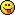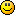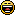# For the puzzlers out there

1. Given: 12 coins, one of which is counterfeit and of a very slightly different weight.  One set of the old fashioned scales; the kind with a pan on each side to put weight on and compare to a weight on the other pan.  You get 3 weighings.  For discussion sake, number the coins 1 to 12; which one is counterfeit?

1. You divide the stack in two. ( I am assuming the conterfeit is either heavier or lighter I am going to assume heavier.)  One stack is weighed against the other.   Pick the stack that is heavier and there will be 6 coins.

Split the stack in half.  Weigh one side against the other.  Pick the heavier stack.  There will be 3 coins.

Weigh 2 coins.  If they are equal in weight the one left out is counterfeit.  If one is heavier than the other that coin is counterfeit.

1. You are not allowed to assume it is heavier - it could be lighter.  You don't know.

2.0
Brenda Durhamposted 13 years agoin reply to this

The one that says Chuck E Cheese on it.1. Now you did it!  You made me spend ALL the coins for pizza!

1.0
woolman60posted 13 years agoin reply to this

Because you do not know what the actual weight, or the true weight of an actual real coin because not all coins weigh the same you can't be sure of selecting the correct coin to apply in the final weighing to compare each coin. Therefore weighing coins in not an option to find the fake coin.

1. If the 11 real coins weigh the same and the one counterfeit is different it is possible.

1. I am missing the trick.  In doing this there are only a couple of ways.  Halving is one way as I had described but then one more weighing is required  to figure out the one that is different.

One could start with 6 coins 3 on each side but the same thing happens and one last weighing is required to determine which one is off.  Starting in the middle is going to produce the least amount of weigh- ins.  Both of these are what would be termed "succesive approximation" which is the most efficient way to do this.

So I need to wait for the trick.

1. No tricks.  One COULD start with 4 against 4 (1/3 of the coins).......

1. Well you got me.  Even 4 against 4, I end up with 4 weighings.  I have tried both replacing coins and swapping them.

I am with Brenda.  I think you should buy us both pizza.

2.0
Brenda Durhamposted 13 years ago

LOL
Yum Pizza!

Well, my brain's no good at mathematical or scientific puzzles.
I'm watching to see if someone actually comes up with the right answer.  Very interesting puzzle!

3. I think we have to swap the coins during the weighings.

1.4. Okay this is a stinker.  I figured it out.

Weigh 1, scenario 1) Weigh 4 against 4.  If both sides are balanced, then the coins that weren't weighed contain the conterfeit.
Weigh 2, scenario 1) Weigh 2 against 2 voila you have it betweent 2 and you can compare weight on the 3rd weigh in.

Weigh 2 scenario 2) When weighing 4 against 4, if one side deflects then we know the coin is contained in the 8.  Swap 3 and which ever side changes action will contain the conterfeit.
At this point we know if the counterfit is heavier or lighter by the initial action of the scale.  Weigh one of the three against the other and note the deflection........If there is a deflection then which ever side has the deflection will be the one that is conterfeit.  If there is no deflection, it is the coin that is left out.

Weigh 2 scenario 3)............There is no deflection among the three coins.  Then it is between 2 coins and one can be compared against another coin to determine which one is conterfeit.

Weight 2 scenario 4.  If there is no deflection, then the pile that is not weighed contains the counterfeit.  One of four on each side is weighed against each other and either causes a delection or not.  The last weighing will just be comparing since it will then be down to 2 coins.This caused me a lot of consternation.  I had to do this on paper because the odd one out does not readily come to mind.

1. IF I understand your actions (words don't always express it well), then wighe 2 scenario 1 weighs 4 coins (one known bad) with 2 on each side.  Right side goes down, but you don't know if it because that side is heavy or the other side is light.  You still have 4 coins, one bad, which you already knew.

weigh 2 scenario 2.  After swapping, if there is no change (say right side down, left up) then the bad coin is one of those not swapped - easy find in one weight left.  But if the action reverses (left side down, right up) then one of the 6 swapped coins is bad, but you still don't know if light or heavy.

Weigh2 scenario 3 - not sure what you're doing.  3 coins on each side?  But which 3 coins?  I got lost

weigh2 scenario - Is it a redo of weigh2 scenario1?  I think I'm lost again.

I found this a fun one - it took over a day to figure it out!

1. This is kind of hard to put into words and I wish I could draw pictures.

I think you are right.

Actually there should be 3 scenarios.  4 & 4 balanced, 4 &4 unbalanced and still unbalanced after 3 are swapped, & 4&4 unbalanced and then balanced after 3 were swapped.

This was a good one.  This one cant be done with linear thinking because we try in our minds to rationalize these by number theory.  However, this needs to be drawn to see what is going on.

Thanks for a good teaser puzzle.

1. I'll post an answer this evening if no one finds it.  It might help to present a picture if each coin is numbered, and each scales pan (left and right) has certain numbered coins on it.  For instance, coins 1,2,3,4 on the left and 5,6,7,8 on the right - result might be right side goes down, left goes up.  Then swap (if I read you right) and put 1,6,7,8 on the left and 5,2,3,4 on the right with a result maybe of left side down and right side up.

I finally had to sit on the floor with 11 pennies and a nickel, moving the nickel around to test the "what if's".  Interestingly, the solution pretty much applies to anything from 3 to 13 coins.

5. I almost got it. I found out how to do the scenario if 4 on 4 coins balance, I just need to figure out how to determine if one is heavier/lighter if they do not balance.

1. That was the easy half!  Had you found the harder half, the easy one would have been obvious.1. Lol yeah it was the easy half XD

6. No takers?  I'll post the answer in a few minutes.

7. OK, no answers so here it is.
Number the coins from 1 to 12 for discussion purposes.  Scales has a left and right side - as one side goes up the other goes down.

Weigh 1   coins 1,2,3,4 on the left, 5,6,7,8 on the right.  Three possibilities; left side up, equal weight, right side up.

Scenario 1    equal weight.  One of the set of 9,10,11,12 is bad, the others are all good.  Weigh 1,2,3 on the left, 9,10,11 on the right.  If even, the bad coin is #12 - if you want the relative weight then weigh it against #1.  If the right side goes down (left side down is analagous solution) then 9,10 or 11 is bad AND heavy.  For the 3rd weighing, 9 on left, 10 on right.  If equal, 11 is a bad coin.  If unequal the side that went down has the bad coin; remember, you already know it is heavy.

Scenario 2  Uneven weight - assume right side down - left down is analagous solution.

Weigh 2  9,10,11,5 on left side, 4,6,7,8 on right side

Scenario 1; equal weight.  The bad coin is either 1,2 or 3 AND is light.  Weigh 1 on left, 2 on right.  If equal, bad coin is #3; if not the bad coin will go up.

Scenario 2:  Unequal weight.  If left side is down, the bad coin is either a light #4 or heavy #5.  For third weighing, put #4 on left and #1 on right.  If unequal, the left side will rise and 4 is the bad coin and is light.  If equal 5 is bad and is heavy.

Secnario 3:  Unequal weight, right side down.  Bad coin is one of 6,7,8 AND is heavy.  Weigh 6 on left and 7 on right; if equal bad coin is #8; if unequal bad coin will go down as it is heavy.

working